Like   Tweet   Pin   +1   in
 /* styles */ We've made it to JUNE!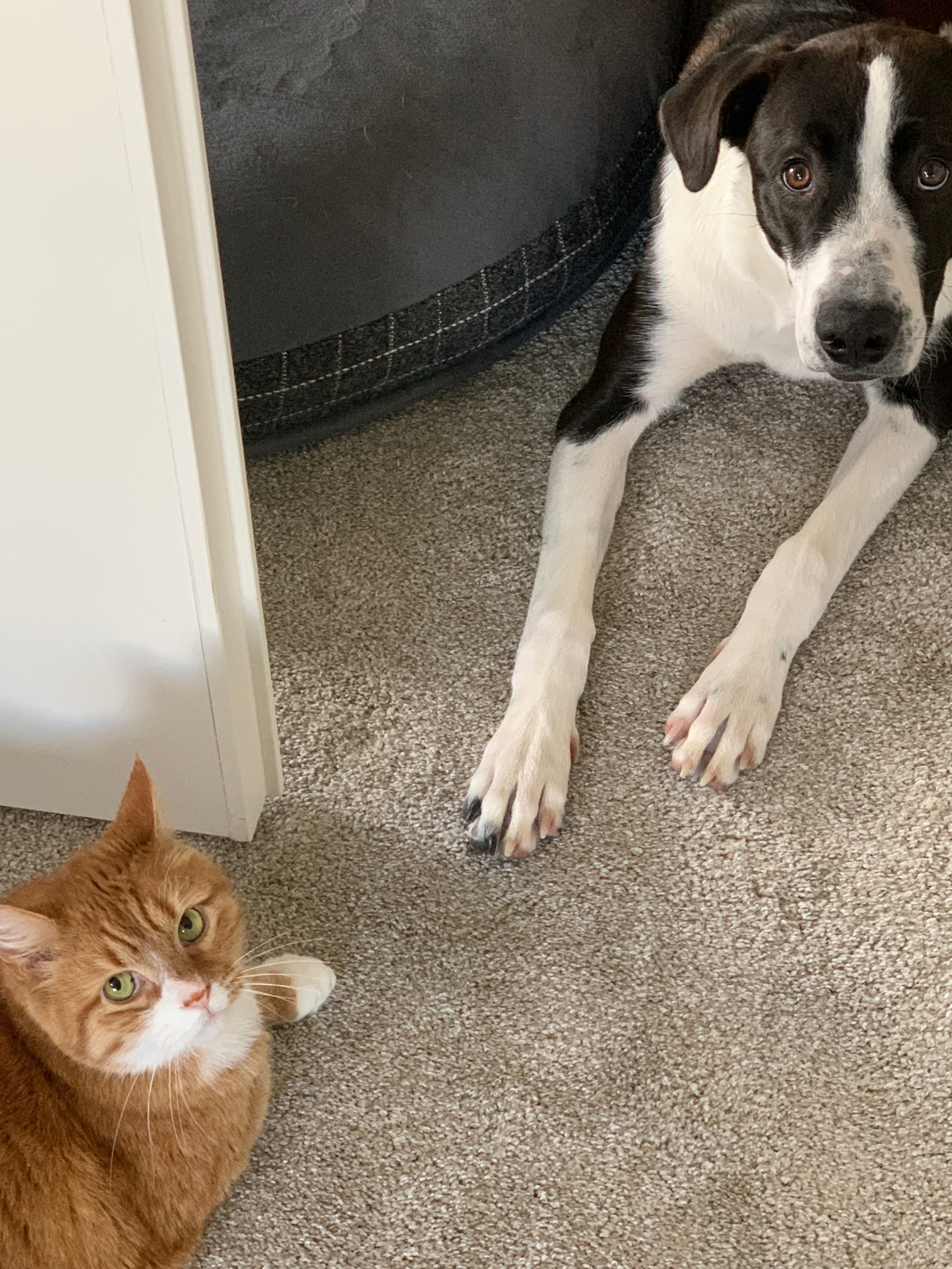How adorable are these two? Chance so badly wants to play with my Juju kitty but Juju can only tolerate Chance for so long. I was super lucky to capture this rafe moment of them laying so close to one another before the chase ensued!

How was your Memorial Day weekend? Ours was pretty low key but enjoyable none-the-less. I was able to get some creating done and I can't wait to show you what I've been up to. Be watching my Social Media accounts for sneak peeks and fun projects coming your was soon!table div table+table+table+table+table+table+table div table td,table.module-6{width:100%;padding:0}table div table+table+table+table+table+table+table div table{width:100%;float:none;margin-left:auto;margin-right:auto;padding:0}table div table+table+table+table+table+table+table div table a{border:0 none;text-decoration:none}table div table+table+table+table+table+table+table div table img{width:100%!important;border:0 none;text-decoration:none}/* styles */
 /* styles */ It’s never been a better time to Join my CTMH Maker family! When you sign up as a new CTMH Maker in June, you’ll receive FREE registration (\$99 value!) for the 2021 Close To My Heart Virtual Convention in July! This is in addition to the 25% savings on all CTMH products you'll start earning! Virtual Convention is a three-day celebration full of crafting, learning, creation, recognition, and connection! As a Convention attendee, you will be among the very first to see and craft with the wealth of exciting new products in our upcoming catalogs!

# NEXT, CLAIM YOUR FREE PAPER PACKET!

## (up to a \$13.95 value!)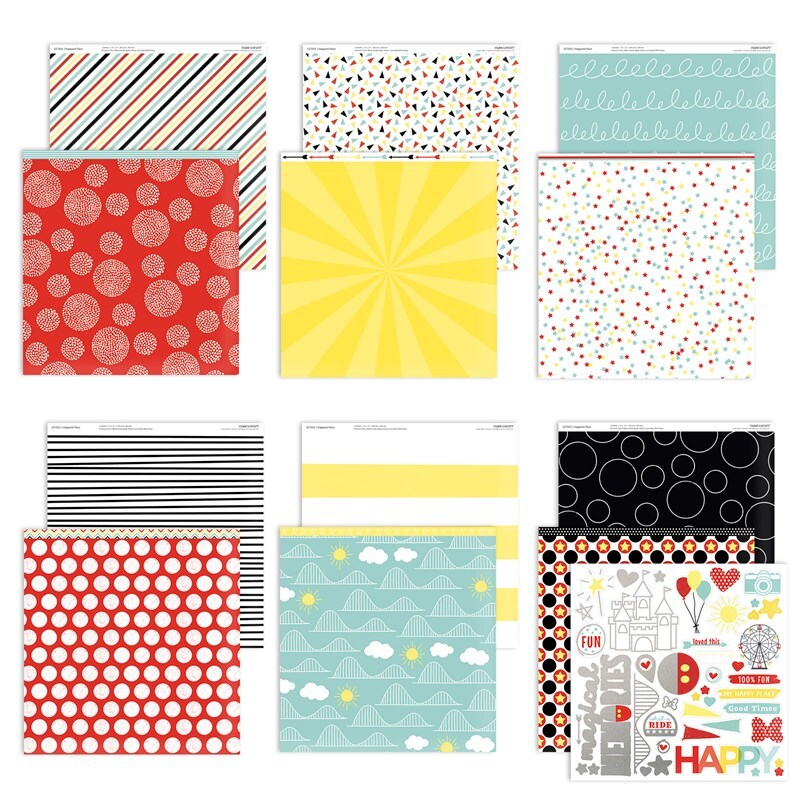/* styles */ When you Join my CTMH Family in June, I will send you ANY Paper Packet of your choosing (up to a \$13.95 value), FREE!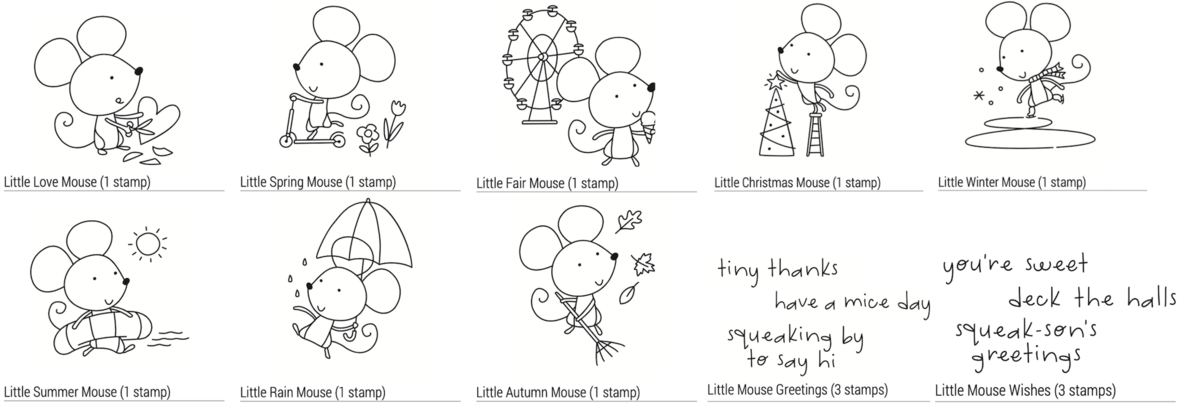/* styles */ START WITH HEART and I'll send you this complete 10-Stamp Set for FREE! - (\$39.50 value!) The Start with Heart program is a 45-day incentive program designed to help new Makers hit the ground running. Research shows that new Makers are more likely to have long-term success when they enjoy immediate achievements. This program helps Makers achieve that success early by offering rewards for reaching certain milestones.# ALSO ON SPECIAL...

 table div table+table+table+table+table+table+table+table+table+table+table+table+table+table+table+table+table+table+table+table div table,table.module-19{width:32.08%;float:left;padding:0}table div table+table+table+table+table+table+table+table+table+table+table+table+table+table+table+table+table+table+table+table div table a{border:0 none;text-decoration:none}table div table+table+table+table+table+table+table+table+table+table+table+table+table+table+table+table+table+table+table+table div table img{width:100%!important;border:0 none;text-decoration:none}table div table+table+table+table+table+table+table+table+table+table+table+table+table+table+table+table+table+table+table+table div table td{width:100%;padding:0 20px 20px 0}/* styles */ RETURN TO THE HAPPIEST PLACE! Relive your happiest memories with the limited time return of the Happiest Place Collection! - I'm so excited! Available through July 31, 2021.
 table div table+table+table+table+table+table+table+table+table+table+table+table+table+table+table+table+table+table+table+table+table div table{width:100%;padding:0}table div table+table+table+table+table+table+table+table+table+table+table+table+table+table+table+table+table+table+table+table+table div table img{width:96.23%;padding:0;float:none}table div table+table+table+table+table+table+table+table+table+table+table+table+table+table+table+table+table+table+table+table+table div table td{width:100%;padding:0 1.88% 18px}/* styles */table div table+table+table+table+table+table+table+table+table+table+table+table+table+table+table+table+table+table+table+table+table+table div table,table.module-21{width:32.08%;float:left;padding:0}table div table+table+table+table+table+table+table+table+table+table+table+table+table+table+table+table+table+table+table+table+table+table div table a{border:0 none;text-decoration:none}table div table+table+table+table+table+table+table+table+table+table+table+table+table+table+table+table+table+table+table+table+table+table div table img{width:100%!important;border:0 none;text-decoration:none}table div table+table+table+table+table+table+table+table+table+table+table+table+table+table+table+table+table+table+table+table+table+table div table td{width:100%;padding:0 20px 20px 0}/* styles */ JUNE STAMP OF THE MONTH! Outdoor Borders - (9 stamps) Each month you can purchase a new D-size Stamp Set (\$18.95 value) for just \$5 - or get it for FREE as a VIP Member! Available through June 30, 2021 only.
 table div table+table+table+table+table+table+table+table+table+table+table+table+table+table+table+table+table+table+table+table+table+table+table div table{width:100%;padding:0}table div table+table+table+table+table+table+table+table+table+table+table+table+table+table+table+table+table+table+table+table+table+table+table div table img{width:96.23%;padding:0;float:none}table div table+table+table+table+table+table+table+table+table+table+table+table+table+table+table+table+table+table+table+table+table+table+table div table td{width:100%;padding:0 1.88% 18px}/* styles */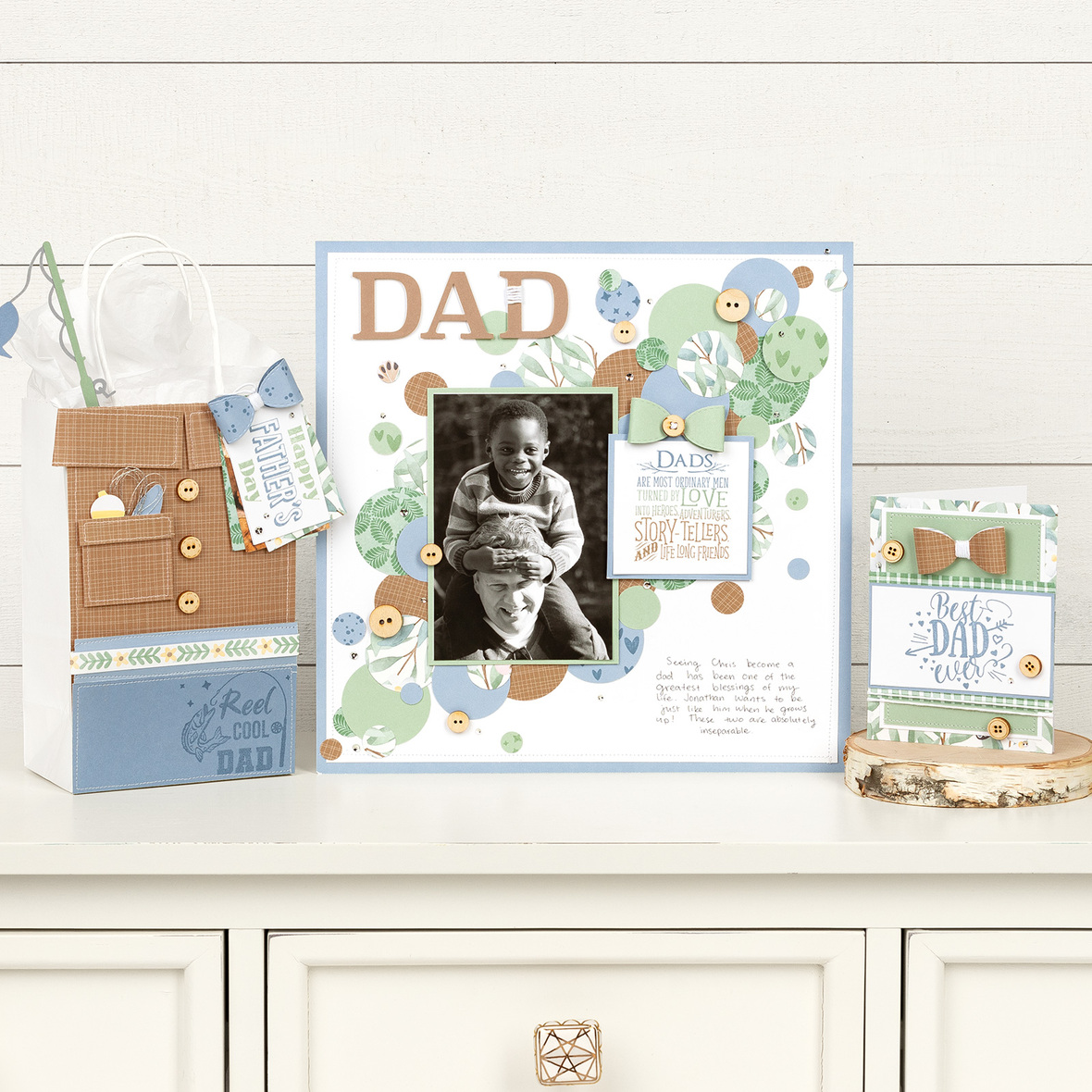Show the dads in your life that you appreciate them using three exclusive stamp sets designed to highlight what makes them special!
Available through June 30, 2021.

 table div table+table+table+table+table+table+table+table+table+table+table+table+table+table+table+table+table+table+table+table+table+table+table+table+table div table{width:100%;padding:0}table div table+table+table+table+table+table+table+table+table+table+table+table+table+table+table+table+table+table+table+table+table+table+table+table+table div table img{width:96.23%;padding:0;float:none}table div table+table+table+table+table+table+table+table+table+table+table+table+table+table+table+table+table+table+table+table+table+table+table+table+table div table td{width:100%;padding:0 1.88% 18px}/* styles */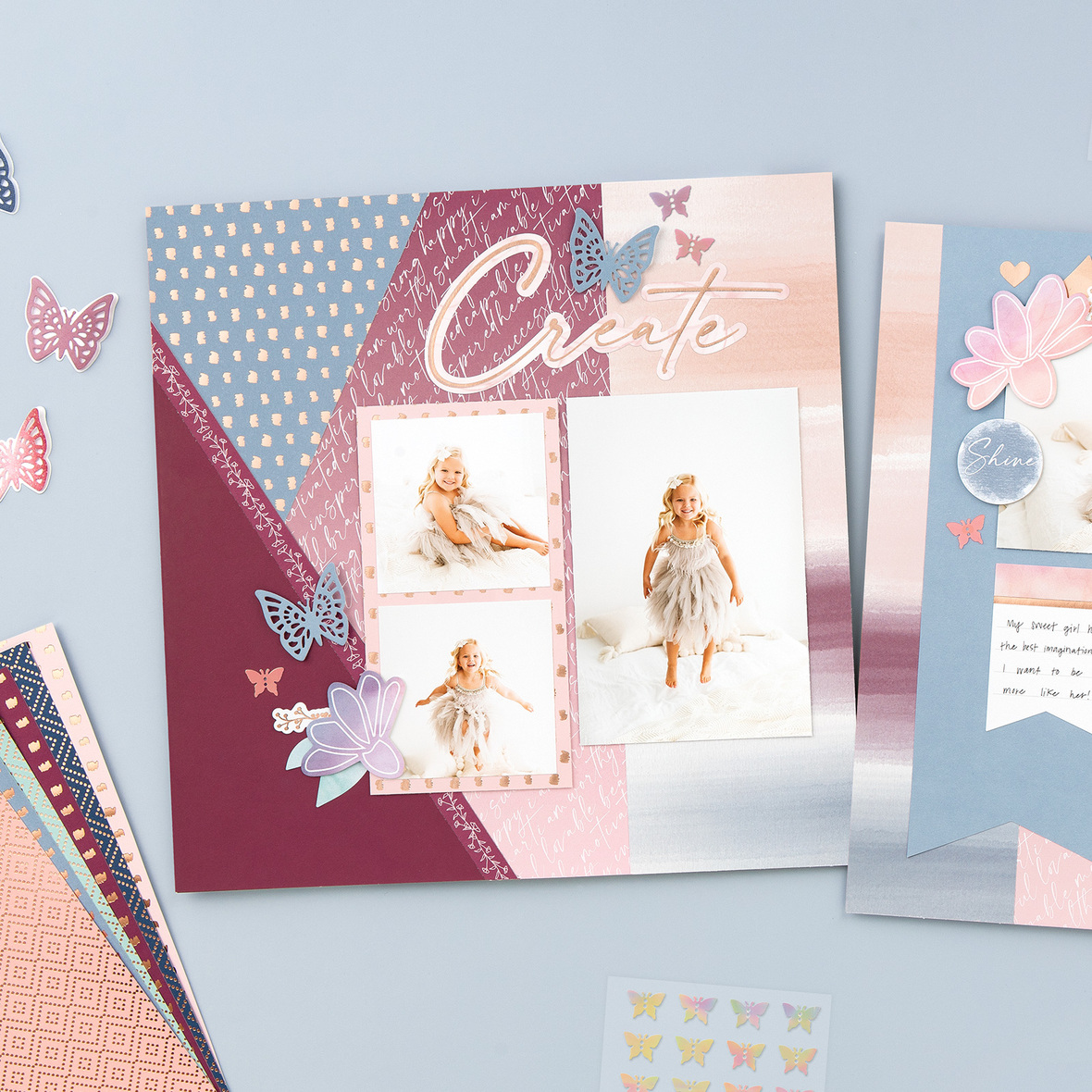YOU ARE ENOUGH
Available through June 30, 2021.## FREE PROCESS VIDEOS!

 table div table+table+table+table+table+table+table+table+table+table+table+table+table+table+table+table+table+table+table+table+table+table+table+table+table+table+table+table+table div table,table.module-28{width:32.08%;float:left;padding:0}table div table+table+table+table+table+table+table+table+table+table+table+table+table+table+table+table+table+table+table+table+table+table+table+table+table+table+table+table+table div table a{border:0 none;text-decoration:none}table div table+table+table+table+table+table+table+table+table+table+table+table+table+table+table+table+table+table+table+table+table+table+table+table+table+table+table+table+table div table img{width:100%!important;border:0 none;text-decoration:none}table div table+table+table+table+table+table+table+table+table+table+table+table+table+table+table+table+table+table+table+table+table+table+table+table+table+table+table+table+table div table td{width:100%;padding:0 20px 20px 0}/* styles */ Just grab the mentioned product (optional) and then mark yourself as 'GOING' in each Facebook event for your chance to win FUN Prizes!

# Here's what's coming up in May:

June 8, 1:00 pm (PST):
Hillside Cottage Cardmaing Workshop - item #G1230

June 21, 1:00pm (PDT):
You Are Enough Cardmaking Workshop - item #CC52119

June 23, 1:00pm (PDT):
You Are Enough Album Workshop - item #CC52121

June 25, 1:00pm (PDT):
You Are Enough Scrapbooking Workshop - item #CC52120

June 29, 1:00pm (PDT):
Hillside Cottage Scrapbooking Workshop - item #G1229/* styles */ If you have any questions, please don't hesitate to ask! I'm always happy to help. Have a fabulous month my Friend!
 table div table+table+table+table+table+table+table+table+table+table+table+table+table+table+table+table+table+table+table+table+table+table+table+table+table+table+table+table+table+table+table+table+table div table td,table.module-32{width:100%;padding:0}table div table+table+table+table+table+table+table+table+table+table+table+table+table+table+table+table+table+table+table+table+table+table+table+table+table+table+table+table+table+table+table+table+table div table{width:100%;float:none;margin-left:auto;margin-right:auto;padding:0}table div table+table+table+table+table+table+table+table+table+table+table+table+table+table+table+table+table+table+table+table+table+table+table+table+table+table+table+table+table+table+table+table+table div table a{border:0 none;text-decoration:none}table div table+table+table+table+table+table+table+table+table+table+table+table+table+table+table+table+table+table+table+table+table+table+table+table+table+table+table+table+table+table+table+table+table div table img{width:100%!important;border:0 none;text-decoration:none}/* styles */
 /* styles */